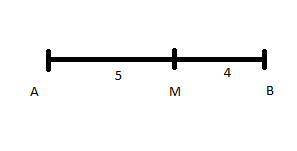QuestionAnswers

# Find the equation of the locus of a point which moves such that the ratio of its distances from$\left( {2,0} \right)$ and $\left( {1,3} \right)$is$5:4$.Verified
129.3k+ views
Hint: Locus of a point is the set of all points that have the same property or we can say the set of points that behaves in the same way. The third theorem of locus states that the locus equidistance from point P and Q is the perpendicular bisector of the line segment joining the two points.
Distance formulae is the method to find the distance between two points S(x, y) and T(x, y) given by the formulae, $D = \sqrt {{{\left( {{x_T} - {x_S}} \right)}^2} + {{\left( {{y_T} - {y_s}} \right)}^2}}$
In the question, the locus of the point is desired such that the ratio of its distances from$\left( {2,0} \right)$ and $\left( {1,3} \right)$ is $5:4$ for which consider a point on the line joining the points $\left( {2,0} \right)$ and $\left( {1,3} \right)$ and at the same time dividing the line segment in the ratio of $5:4$ and use the distance formula accordingly.

Let us consider the point$M(x,y)$which lies between the point $A\left( {2,0} \right)$and$B\left( {1,3} \right)$, the point $M(x,y)$divides the line into $5:4$ratio,Now find the distance of point M from point A and from point B by using the distance formula
${d_{MA}} = \sqrt {{{\left( {{x_M} - {x_A}} \right)}^2} + {{\left( {{y_M} - {y_A}} \right)}^2}} \\ = \sqrt {{{\left( {x - 2} \right)}^2} + {{\left( {y - 0} \right)}^2}} \\ = \sqrt {{{\left( {x - 2} \right)}^2} + {{\left( y \right)}^2}} \\$
And the distance from B
${d_{MB}} = \sqrt {{{\left( {{x_M} - {x_B}} \right)}^2} + {{\left( {{y_M} - {y_B}} \right)}^2}} \\ = \sqrt {{{\left( {x - 1} \right)}^2} + {{\left( {y - 3} \right)}^2}} \\$
Given that the point $M(x,y)$divides the line AB into$5:4$ratio, hence we can write
$\dfrac{{{d_{MA}}}}{{{d_{MB}}}} = \dfrac{5}{4}$
This is equal to
$\dfrac{{{d_{MA}}}}{{{d_{MB}}}} = \dfrac{5}{4} \\ \dfrac{{\sqrt {{{\left( {x - 2} \right)}^2} + {{\left( y \right)}^2}} }}{{\sqrt {{{\left( {x - 1} \right)}^2} + {{\left( {y - 3} \right)}^2}} }} = \dfrac{5}{4} \\ \dfrac{{{{\left( {x - 2} \right)}^2} + {y^2}}}{{{{\left( {x - 1} \right)}^2} + {{\left( {y - 3} \right)}^2}}} = \dfrac{{{5^2}}}{{{4^2}}} \\ \dfrac{{{{\left( {x - 2} \right)}^2} + {y^2}}}{{{{\left( {x - 1} \right)}^2} + {{\left( {y - 3} \right)}^2}}} = \dfrac{{25}}{{16}} \\$
Now use the cross multiplication to solve the equation further, hence we can write
$16\left\{ {{{\left( {x - 2} \right)}^2} + {y^2}} \right\} = 25\left\{ {{{\left( {x - 1} \right)}^2} + {{\left( {y - 3} \right)}^2}} \right\} \\ 16\left\{ {{x^2} - 4x + 4 + {y^2}} \right\} = 25\left\{ {{x^2} - 2x + 1 + {y^2} - 6y + 9} \right\} \\ 16{x^2} - 64x + 16{y^2} + 64 = 25{x^2} - 50x + 25 + 25{y^2} - 150y + 225 \\ 9{x^2} + 9{y^2} + 14x - 150y + 186 = 0 \\$
Hence we get the equation of the locus at the point $M(x,y)$equal to $9{x^2} + 9{y^2} + 14x - 150y + 186 = 0$

Note: Locus can be a set of points, lines, line segments, curve, and surface which satisfy one or more properties. If the locus of the point divides the line between two points in the ratio $1:1$then the point locus is equidistant from both the points.